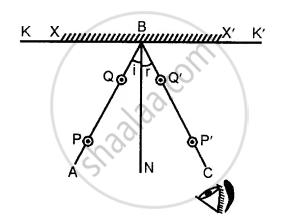ICSE Class 7CISCE
Share

# Describe an Experiment to Verify the Laws of Reflection of Light. Ans. Laws of Reflection. - ICSE Class 7 - Physics

ConceptLaws of Reflection

#### Question

Describe an experiment to verify the laws of reflection of light. Ans. Laws of reflection.

#### Solution

(i) The incident ray, the reflected ray and the normal at the point of incidence, lie in the same plane.
(ii) The angle of incidence and angle of reflection are equal i.e. ∠i = ∠r.
Verification
Take a wooden drawing board and fix a white sheet of paper on it. In the middle of paper draw a straight line KK\ Mark a point B on it. Draw a perpendicular BN. Place a mirror XX’ on line KK’ such that polished side of mirror is along the line. Hold-the mirror in the mirror holder.Fix two steel pins P and Q on the straight line AB atleast 10 cm apart. Look for the images of the pins P and Q and fix two pins P Q’ such that P’, Q’ and images of P and Q are all in the same straight line. Remove the pins and draw small circles around the pin pricks.
Remove the mirror also. Join P’Q’ and produce the straight line to meet at B.
Measure ∠ABN = i and ∠CBN = r.
It is found that ∠i = ∠r. This proves that Angle of Incidence is equal to Angle of Reflection.
As the incident ray, the reflected ray and the normal lie in the plane of paper, therefore, they lie in the same plane.

Is there an error in this question or solution?
Solution Describe an Experiment to Verify the Laws of Reflection of Light. Ans. Laws of Reflection. Concept: Laws of Reflection.
S